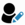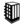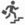Core Java - Interview Questions and Answers for 'Recursion]' | Search Java Interview Question - javasearch.buggybread.com# Search Interview QuestionsMore than 3000 questions in repository.There are more than 900 unanswered questions.Have a video suggestion.
Click Correct / Improve and please let us know.
Label / Company      Label / Company / TextSubmit Question

Core Java - Interview Questions and Answers for 'Recursion]' - 7 question(s) found - Order By NewestQ1. Write a program to calculate factorial of a number using recursionCore JavaAns.

public class Factorial {
public static void main(String[] args){
int x = 5;

System.out.println(calculateFactorial(x));

}

private static int calculateFactorial(int x){
if(x==1){
return 1;
}
return x * calculateFactorial(x-1);
}
}Help us improve. Please let us know the company, where you were asked this question :LikeDiscussCorrect / Improvefactorial  calculate factorial  code  coding  recursionAsked in 1 CompaniesbasicQ2. Write an Algorithm for Graph Traversal ? The Graph has a loop.Algorithm2016-11-30 15:42:32Ans. Please not that all such questions can be easily answered through recursion.

Simple recursive implementation could be

traverse(root);

void traverse(Element element){
if(element.hasNext()){
traverse(element.next());
} else {
System.out.println(element);
}
}

but this algo / code lead to endless loop if there is a loop in graph traversal.

So you can keep a collection to keep track of which elements have laready been traversed

static List<Elements> listOfAlreadyTraversedElements = new ArrayList<Elements>();

main(){
traverse(root);
}

void traverse(Element element){
if(element.hasNext()){
traverse(element.next());
} else {
System.out.println(element);
}
}Help us improve. Please let us know the company, where you were asked this question :LikeDiscussCorrect / Improvegraph traversal algorithm  graph traversal algorithm using recursionAsked in 1 CompaniesintermediateQ3. Write a Program to print factorial of a number using recursionCore Java2017-01-29 17:47:20public static void main(String args[]) {
System.out.println(factorial(5));
}

private static int factorial(int number){
if(number == 1){
return 1;
} else {
return number * factorial(number - 1);
}
}
}Help us improve. Please let us know the company, where you were asked this question :LikeDiscussCorrect / ImproverecursionAsked in 24 CompaniesQ4. Write a program to print sum of numbers between the start and end number

For example - Passing start number as 2 and end number as 10, it should print 2+3+4+5+6+7+8+9+10 = 54
Core Java2017-01-29 17:52:10Ans.
public static void main(String args[]) {
System.out.println(sum(2,10));
}

private static int sum(int start,int end){
if(start > end){
return 0;
} else {
return start + sum(start+1,end);
}
}
}Help us improve. Please let us know the company, where you were asked this question :LikeDiscussCorrect / ImproverecursionQ5. Write a program using Recursion to print multiplication of numbers between the start and end number

For example - Passing start number as 2 and end number as 10, it should print 2*3*4*5*6*7*8*9*10 = 3628800
Core Java2017-01-29 17:54:47Ans.
public static void main(String args[]) {
System.out.println(multiply(2,5));
}

private static int multiply(int start,int end){
if(start > end){
return 1;
} else {
return start * multiply(start+1,end);
}
}
}Help us improve. Please let us know the company, where you were asked this question :LikeDiscussCorrect / ImproverecursionQ6. Which of the two - iteration or recursion - is slower ?Core Java2017-08-10 17:03:28Ans. Any function call is slower than the code in the same method as Java has to maintain stack of meta and function call information. So recursion is slower.Help us improve. Please let us know the company, where you were asked this question :LikeDiscussCorrect / Improveiteration  recursion  iteration vs recursionQ7. Write a Program to print fibonacci series using recursion.Core Java2017-08-24 14:41:45Ans. public class FibonacciUsingRecursion {
public static void main(String[] args) throws IOException{
getNextFibanocci(1,1);
}

static int getNextFibanocci(int a,int b){
if(a+b >= 100){
return 0;
} else {
System.out.println(a+b);
getNextFibanocci(b,a+b);
}

return 0;
}
}Help us improve. Please let us know the company, where you were asked this question :LikeDiscussCorrect / Improvefibonacci  recursion

## Help us and Others Improve. Please let us know the questions asked in any of your previous interview.

Any input from you will be highly appreciated and It will unlock the application for 10 more requests.